# Out-of-Distribution Data Discriminator for YOLO v4 Object Detector

This example shows how to detect out-of-distribution (OOD) data in a YOLO v4 object detector.

OOD data detection is the process of identifying inputs to a deep neural network that might yield unreliable predictions. OOD data refers to data that is different from the data used to train the model. For example, data collected in a different way, under different conditions, or for a different task than the data on which the model was originally trained.

By assigning confidence scores to the predictions of a network, you can classify data as in-distribution (ID) or OOD. You can then choose how you treat OOD data. For example, you can choose to reject the prediction of a neural network if the network detects OOD data.

This example shows how to train a model to detect vehicles in images and construct a discriminator to classify the images as ID or OOD.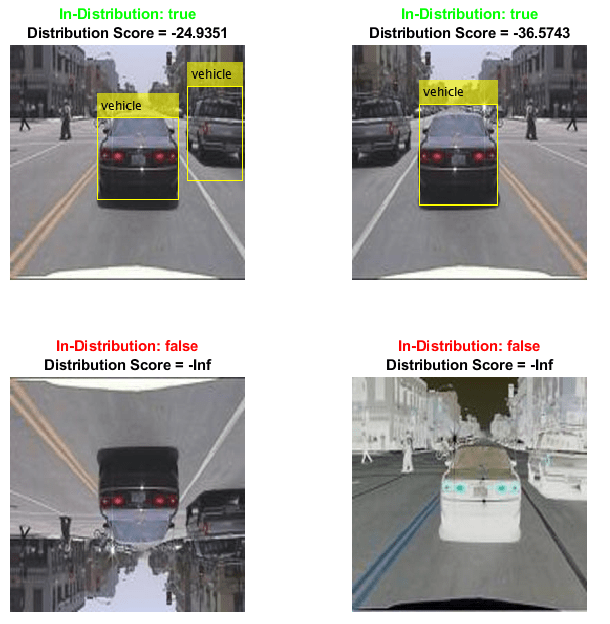Note: This example requires the Computer Vision Toolbox™ Model for YOLO v4 Object Detection and the Deep Learning Toolbox™ Verification Library support packages. You can install these add-ons from Add-On Explorer. For more information about installing add-ons, see Get and Manage Add-Ons. Alternatively, see Deep Learning Toolbox Verification Library.

### Prepare Training Data

This example uses a small vehicle data set that contains 295 images. Many of these images come from the Caltech Cars 1999 and 2001 data sets, created by Pietro Perona and used with permission. Each image contains one or two labeled instances of a vehicle. A small data set is useful for exploring the YOLO v4 training procedure, but in practice, more labeled images are needed to train a robust detector.

Unzip the vehicle images and load the vehicle ground truth data.

```unzip vehicleDatasetImages.zip data = load('vehicleDatasetGroundTruth.mat'); vehicleDataset = data.vehicleDataset;```

Add the full path to the local vehicle data folder.

`vehicleDataset.imageFilename = fullfile(pwd,vehicleDataset.imageFilename);`

Split the data set into training and validation sets. Use 60% of the data for training and 40% for validation.

```rng("default"); shuffledIndices = randperm(height(vehicleDataset)); idx = floor(0.6 * length(shuffledIndices)); trainingDataTbl = vehicleDataset(shuffledIndices(1:idx),:); validationDataTbl = vehicleDataset(shuffledIndices(idx+1:end),:);```

Use `imageDatastore` and `boxLabelDatastore` (Computer Vision Toolbox) to create datastores for loading the image and label data during training and validation.

```imdsTrain = imageDatastore(trainingDataTbl.imageFilename); bldsTrain = boxLabelDatastore(trainingDataTbl(:,"vehicle")); imdsValidation = imageDatastore(validationDataTbl.imageFilename); bldsValidation = boxLabelDatastore(validationDataTbl(:,"vehicle"));```

Combine the image and box label datastores.

```dsTrain = combine(imdsTrain,bldsTrain); dsValidation = combine(imdsValidation,bldsValidation); numObservationsTrain = numel(imdsTrain.Files); numObservationsValidation = numel(imdsValidation.Files);```

Specify the input size to use for resizing the images and the bounding boxes. For the pretrained YOLO v4 detector, the underlying base networks require the size of the training images to be a multiple of 32.

`inputSize = [224 224 3];`

### Estimate Anchor Boxes

Use the `estimateAnchorBoxes` (Computer Vision Toolbox) function to estimate anchor boxes based on the size of objects in the training data. To account for the resizing of the images prior to training, resize the training data for estimating anchor boxes. Use the `transform` function to preprocess the training data, then define the number of anchor boxes and estimate the anchor boxes. Resize the training data to the input size of the network by using the `preprocessData` helper function.

```trainingDataForEstimation = transform(dsTrain,@(data)preprocessData(data,inputSize)); numAnchors = 6; [anchors,meanIoU] = estimateAnchorBoxes(trainingDataForEstimation,numAnchors); area = anchors(:,1).*anchors(:,2); [~,idx] = sort(area,"descend"); anchors = anchors(idx,:); anchorBoxes = {anchors(1:3,:);anchors(4:6,:)};```

For more information about choosing anchor boxes, see Estimate Anchor Boxes From Training Data (Computer Vision Toolbox) and Anchor Boxes for Object Detection (Computer Vision Toolbox).

### Configure and Train YOLO v4 Network

This example uses a YOLO v4 network pretrained on the COCO data set. In this example, you fine-tune the detector for detecting vehicles in an image. For more information on the fine-tuning and the vehicle data set used for the fine-tuning, see `trainYOLOv4ObjectDetector` (Computer Vision Toolbox).

Specify the class names and configure the pretrained YOLO v4 deep learning network for the new data set by using the `yolov4ObjectDetector` (Computer Vision Toolbox) function.

```classes = "vehicle"; detector = yolov4ObjectDetector("tiny-yolov4-coco",classes,anchorBoxes,InputSize=inputSize);```

Specify the training options and retrain the pretrained YOLO v4 network on the new data set by using the `trainYOLOv4ObjectDetector` function.

```options = trainingOptions("sgdm", ... InitialLearnRate=0.001, ... MiniBatchSize=16, ... MaxEpochs=50, ... ValidationData=dsValidation, ... BatchNormalizationStatistics="moving", ... ResetInputNormalization=false, ... VerboseFrequency=30);```

To save time, set the `doTraining` flag to `false` and load a pretrained network. If you want to train the detector, set the `doTraining` value to `true`.

```doTraining = false; if doTraining trainedDetector = trainYOLOv4ObjectDetector(dsTrain,detector,options); else filename = matlab.internal.examples.downloadSupportFile("nnet","data/trainedYolov4VehicleDetectionNetwork.mat"); load(filename); end```

Examine the underlying backbone network for the YOLO v4 detector. For more information, see Getting Started with YOLO v4 (Computer Vision Toolbox).

`net = trainedDetector.Network`
```net = dlnetwork with properties: Layers: [74×1 nnet.cnn.layer.Layer] Connections: [80×2 table] Learnables: [80×3 table] State: [38×3 table] InputNames: {'input_1'} OutputNames: {'convOut1' 'convOut2'} Initialized: 1 View summary with summary. ```

### Detect Vehicles in Image

```reset(dsTrain) imgIdx = 1; img = read(dsTrain); img = img{imgIdx};```

Normalize and resize the image.

```img = im2single(img); img = imresize(img,inputSize(1:2));```

Detect vehicles and find the bounding boxes in the sample image.

```detectorThr = 0.05; [bboxes,~,labels] = detect(trainedDetector,img,Threshold=detectorThr); detectedImage = insertObjectAnnotation(img,"Rectangle",bboxes,labels);```

Display the image and bounding boxes.

```figure imshow(detectedImage)```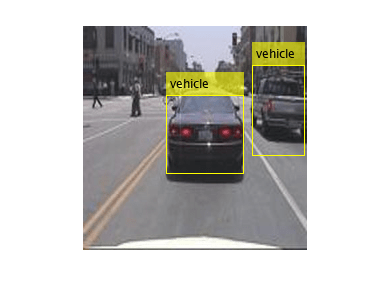### Test Object Detector on OOD Data

The network can detect objects in images like those on which it was trained. Test how the network performs on data that you did not use during training.

Augment the image to generate images different to those on which the network was trained. Use three types of image augmentation to generate the images:

• Flip the image in the left-right direction.

• Flip the image in the up-down direction.

• Invert the image.

```imgLRFlip = fliplr(img); imgUDFlip = flipud(img); imgInvert = 1-img;```

Use the fine-tuned YOLO v4 object detector to detect vehicles in the three augmented images.

```[bboxesLRFlip,~,labelsLRFlip] = detect(trainedDetector,imgLRFlip,Threshold=detectorThr); detectedLRFlip = insertObjectAnnotation(imgLRFlip,"Rectangle",bboxesLRFlip,labelsLRFlip); [bboxesUDFlip,~,labelsUDFlip] = detect(trainedDetector,imgUDFlip,Threshold=detectorThr); detectedUDFlip = insertObjectAnnotation(imgUDFlip,"Rectangle",bboxesUDFlip,labelsUDFlip); [bboxesInvert,~,labelsInvert] = detect(trainedDetector,imgInvert,Threshold=detectorThr); detectedInvert = insertObjectAnnotation(imgInvert,"Rectangle",bboxesInvert,labelsInvert);```

Display the results for the original image and the augmented images using the helper function `plotAugmentedImages`, found at the end of this example. The network is unable to detect the car in the up-down flipped and the inverted images.

```figure plotAugmentedImages( ... detectedImage,"Original", ... detectedLRFlip,"Left-Right Flipped", ... detectedUDFlip,"Up-Down Flipped", ... detectedInvert,"Pixel Inverted")```When deploying models to safety-critical environments, it is important to have a method of assigning confidence to the network predictions. It is also important to have a method of classifying the image as outside of the distribution of data that the model has been trained on.

### Create OOD Data Discriminator

You can assign confidence to network predictions by computing a distribution confidence score for each observation. ID data usually has a higher confidence score than OOD data . You can then apply a threshold to the scores to determine whether an input is ID or OOD. The discriminator acts as an additional output of the trained network which classifies an observation as ID or OOD.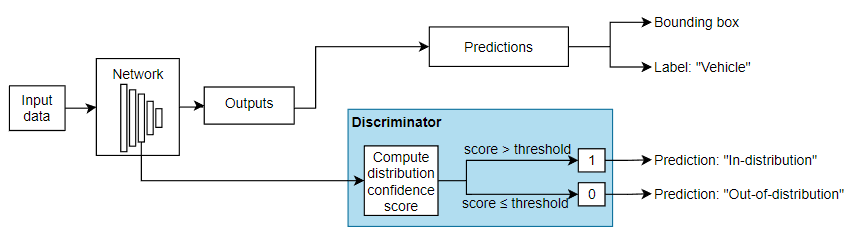You can use the `networkDistributionDiscriminator` function to create a discriminator to separate data into ID and OOD. The function returns a discriminator containing a threshold for separating data into ID and OOD using their distribution scores.

To create a discriminator for a network with multiple outputs, you must have a set of ID data. The ID data must also be a `dlarray` object. In this example, you use the training data to create a data discriminator. Use the helper function `convertToDlarray`, found at the end of this example, to convert the data to a `dlarray` object. The helper function normalizes the data and resizes it to the input size the network expects. The function then returns a `dlarray` object. For more information about image preprocessing, see `trainYOLOv4ObjectDetector` (Computer Vision Toolbox).

`XTrain = convertToDlarray(dsTrain,numObservationsTrain);`

Using the `networkDistributionDiscriminator` function and the training data as the ID data, create a distribution discriminator object using the histogram based outlier scores (HBOS) method  with a true positive goal of 0.95. The function creates a discriminator object containing a threshold for separating the ID and OOD data and a method for computing confidence scores. The software chooses a threshold such that at least 95% of the distribution confidence scores for the ID data are above the threshold. By default, the function computes the distribution scores using first output layer (`'convOut1'`). The HBOS method calculates distribution scores by modeling the underlying features of the network using histograms. For more information, see Distribution Confidence Scores.

`discriminator = networkDistributionDiscriminator(net,XTrain,[],"hbos")`
```discriminator = HBOSDistributionDiscriminator with properties: Method: "hbos" Network: [1×1 dlnetwork] LayerNames: "convOut1" VarianceCutoff: 1.0000e-03 Threshold: -42.4617 ```

Find the threshold for separating ID and OOD data.

`discriminatorThreshold = discriminator.Threshold`
```discriminatorThreshold = single -42.4617 ```

### Examine Feature Dependence

The HBOS algorithm assumes that the features are statistically independent when constructing univariate histograms for scoring. You can test this assumption using statistical techniques. For example, if you have the Statistics and Machine Learning Toolbox™, then you can test the degree of pairwise rank correlation of the features using the `corr` (Statistics and Machine Learning Toolbox) function. To test for feature dependence, set the `doDependenceTest` flag to `true`.

```doDependenceTest = true; if doDependenceTest```

Use the `principalComponentFeatureCorrelation` supporting function to compute the correlation between each pair of features and the p-value. You can use the p-value to test the hypothesis of no correlation against the alternative hypothesis of a nonzero correlation. If `p(a,b)` is small (less than `0.05`), then the correlation `rho(a,b)` is significantly different from zero.

` [rho,p] = principalComponentFeatureCorrelation(discriminator,XTrain);`

Plot the surface of correlation coefficients.

``` figure heatmap(rho,GridVisible="off",ColorLimits=[0,1],Title="Pairwise Feature Correlation")```

Specify a significance level of `0.05`. For multiple comparisons, use the Bonferroni correction to test the hypothesis that the pairwise correlations between any two principal component features is zero. If for a given pair of features, p is less than the significance divided by the correction, then reject the hypothesis that those features have zero correlation. Plot the surface showing pairs of principal component features that reject the hypothesis of zero correlation.

``` pvalue = 0.05; numFeatures = size(p,1); bonferroniCorrection = numFeatures*(numFeatures-1)/2; rejectZeroCorrHypotheses = p < pvalue / bonferroniCorrection; figure heatmap(single(rejectZeroCorrHypotheses),GridVisible="off",ColorLimits=[0,1],Title="Reject Zero Correlation Hypothesis")```

In this example, the hypothesis that no correlation exists between any two features is not rejected.

`end`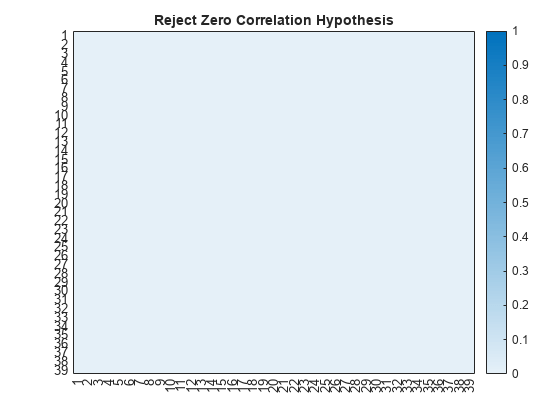### Test OOD Data Discriminator

Test the performance of the discriminator on the augmented training data.

`XTrain = convertToDlarray(dsTrain,numObservationsTrain);`

Generate OOD data sets by augmenting each training image.

```XTrainLRFlip = fliplr(XTrain); XTrainUDFlip = flipud(XTrain); XTrainInvert = 1-XTrain;```

Find the distribution scores for each of the data sets using the discriminator.

```scoresTrain= distributionScores(discriminator,XTrain); scoresTrainLRFlip = distributionScores(discriminator,XTrainLRFlip); scoresTrainUDFlip = distributionScores(discriminator,XTrainUDFlip); scoresTrainInvert = distributionScores(discriminator,XTrainInvert);```

Find the receiver operating characteristic (ROC) curves for the original and augmented data sets using the discriminator and the distribution scores. A well-performing discriminator achieves an AUC value close to 1, corresponding to the discriminator being able to separate the ID and OOD data.

```trueDataLabels = [ repelem("Training",numObservationsTrain), ... repelem("Augmented",numObservationsTrain)]; scoresTrainAndLRFlip = [scoresTrain',scoresTrainLRFlip']; scoresTrainAndUDFlip = [scoresTrain',scoresTrainUDFlip']; scoresTrainAndInvert = [scoresTrain',scoresTrainInvert']; rocObjTrainLRFlip = rocmetrics(trueDataLabels,gather(scoresTrainAndLRFlip),"Training"); rocObjTrainUDFlip = rocmetrics(trueDataLabels,gather(scoresTrainAndUDFlip),"Training"); rocObjTrainInvert = rocmetrics(trueDataLabels,gather(scoresTrainAndInvert),"Training");```

Plot the ROC curves. If the discriminator performs well, then the ROC curve should be close to the top-left corner (corresponding to an AUC value of 1). The ROC curves show that the discriminator can distinguish the augmented images (OOD) from the training images (ID). The discriminator has greatest difficulty distinguishing the left-right flipped images as OOD. This is because those images are closer to the distribution of the training images. To ensure the model is robust to these augmentations, retrain the model using the augmented images. For more information, see Object Detection Using YOLO v4 Deep Learning (Computer Vision Toolbox).

```figure tiledlayout(2,2) nexttile plot(rocObjTrainLRFlip,ShowModelOperatingPoint=false) title("Left-Right Flipped") nexttile plot(rocObjTrainUDFlip,ShowModelOperatingPoint=false) title("Up-Down Flipped") nexttile plot(rocObjTrainInvert,ShowModelOperatingPoint=false) title("Pixel Inverted")```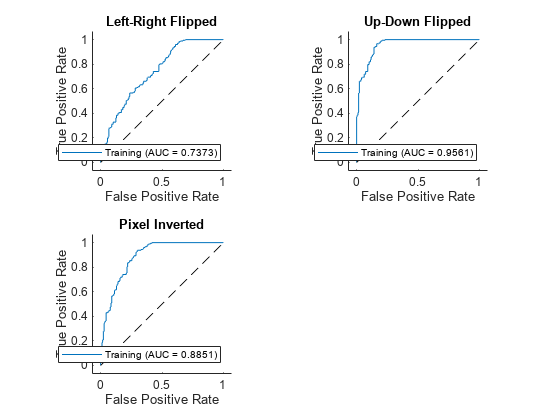The discriminator selects a threshold that best splits the ID and OOD data. Use the `isInNetworkDistribution` function to see the proportion of images classified as OOD by the discriminator. The function returns `1` (`true`) if the discriminator classifies the image as ID and `0` (`false`) if the discriminator classifies the image as OOD.

Test the discriminator on the original training data.

```tfTrain = isInNetworkDistribution(discriminator,XTrain); propotionTrainID = sum(tfTrain)/numel(tfTrain)```
```propotionTrainID = 0.9548 ```

For the training data, the proportion of ID observations is above the true positive goal of 0.975 specified when creating the discriminator.

Test the discriminator on the augmented training data. Find the proportion of left-right flipped images that the network classifies as ID.

```tfTrainLRFlip = isInNetworkDistribution(discriminator,XTrainLRFlip); propotionTrainLRFlipID = sum(tfTrainLRFlip)/numel(tfTrainLRFlip)```
```propotionTrainLRFlipID = 0.6045 ```

Find the proportion of up-down flipped images that the network classifies as ID.

```tfTrainUDFlip = isInNetworkDistribution(discriminator,XTrainUDFlip); propotionTrainUDFlipID = sum(tfTrainUDFlip)/numel(tfTrainUDFlip)```
```propotionTrainUDFlipID = 0.1695 ```

Find the proportion of inverted images that the network classifies as ID.

```tfTrainInvert= isInNetworkDistribution(discriminator,XTrainInvert); propotionTrainInvertID = sum(tfTrainInvert)/numel(tfTrainInvert)```
```propotionTrainInvertID = 0.3503 ```

Visualize the results for the first image. The discriminator classifies the original training and the left-right flipped image as ID. The left-right flipped image is similar to the images that the network sees during training, so it is not surprising that the discriminator classifies this as ID. The left-right flipped image has a lower distribution confidence score reflecting the additional uncertainty. The up-down flipped and inverted images have a distribution score of `-Inf`. This is because those images are outside of the range of histograms that the HBOS method uses to compute the scores.

```figure tiledlayout(2,2) nexttile imshow(detectedImage) colorTitle(tfTrain(1),scoresTrain(1)) nexttile imshow(detectedLRFlip) colorTitle(tfTrainLRFlip(1),scoresTrainLRFlip(1)) nexttile imshow(detectedUDFlip) colorTitle(tfTrainUDFlip(1),scoresTrainUDFlip(1)) nexttile imshow(detectedInvert) colorTitle(tfTrainInvert(1),scoresTrainInvert(1))```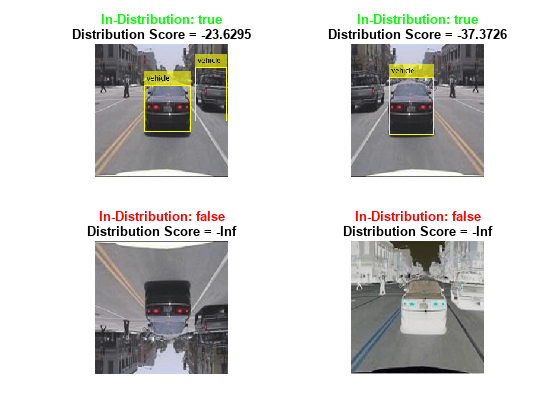### References

 Shalev, Gal, Gabi Shalev, and Joseph Keshet. “A Baseline for Detecting Out-of-Distribution Examples in Image Captioning.” In Proceedings of the 30th ACM International Conference on Multimedia, 4175–84. Lisboa Portugal: ACM, 2022. https://doi.org/10.1145/3503161.3548340.

 Markus Goldstein and Andreas Dengel. "Histogram-based outlier score (hbos): A fast unsupervised anomaly detection algorithm." KI-2012: poster and demo track 9 (2012).

### Helper Functions

#### `preprocessData`

`The preprocessData` function takes as input data containing the image and the bounding box, and a target size, and returns the image and bounding box resized to match the target size.

```function data = preprocessData(data,targetSize) for num = 1:size(data,1) I = data{num,1}; imgSize = size(I); bboxes = data{num,2}; I = im2single(imresize(I,targetSize(1:2))); scale = targetSize(1:2)./imgSize(1:2); bboxes = bboxresize(bboxes,scale); data(num,1:2) = {I,bboxes}; end end```

#### `preprocessMiniBatch`

The `preprocessMiniBatch` function preprocesses a mini-batch of data by extracting the image data from the input cell array and concatenating the data into a numeric array.

```function x = preprocessMiniBatch(xCell,~,~) targetSize = [224 224 3]; for num = 1:size(xCell,1) I = xCell{num,1}; I = im2single(imresize(I,targetSize(1:2))); xCell{num,1} = I; end % Concatenate over batch. x = cat(4,xCell{:}); end```

#### `convertToDlarray`

The `convertToDlarray` function takes as input a datastore and returns the data as a `dlarray` object.

```function X = convertToDlarray(ds,numObservations) minibatchsize = numObservations; numOutputs = 1; mbq = minibatchqueue(ds,numOutputs,... MiniBatchSize=minibatchsize,... MiniBatchFcn=@preprocessMiniBatch, ... OutputAsDlarray=ones(1,numOutputs), ... MiniBatchFormat="SSCB"); X = next(mbq); end```

#### `plotAugmentedImages`

Th `plotAugmentedImages` function takes as input four pairs of images (`x`, `y`, `z`, and `w`) and image titles (`xtitle`, `ytitle`, `ztitle`, and `wtitle`) and returns a plot.

```function plotAugmentedImages(x,xtitle,y,ytitle,z,ztitle,w,wtitle) tiledlayout(2,2) nexttile imshow(x) title(xtitle) nexttile imshow(y) title(ytitle) nexttile imshow(z) title(ztitle) nexttile imshow(w) title(wtitle) end```

#### `colorTitle`

The `colorTitle` function takes as input a logical value indicating if the image is ID, and the raw `score`, and returns a custom figure title.

```function colorTitle(tf,score) if gather(tf) title({"\color{green}" + "In-Distribution: " + gather(tf) + "\color{black}"; "Distribution Score = " + gather(score)}) else title({"\color{red}" + "In-Distribution: " + gather(tf) + "\color{black}"; "Distribution Score = " + gather(score)}) end end```

#### `principalComponentFeatureCorrelation`

The `principalComponentFeatureCorrelation` function takes as input a discriminator object and ID data and returns a matrix of the pairwise correlation coefficient between each pair of features and the p-values.

```function [rho,p] = principalComponentFeatureCorrelation(discriminator,X) layerName = discriminator.LayerNames; features = predict(discriminator.Network,X,Outputs=layerName); % Flatten the SSC channels. nonBatchDims = [finddim(features,"S") finddim(features,"C")]; batchDim = finddim(features,"B"); sz = size(features); features = reshape(extractdata(gather(features)), ... [prod(sz(nonBatchDims)),prod(sz(batchDim))]); features = features'; % Compute the principal components. [coeff,~,latent] = pca(features); rank = sum( latent/max(latent) > discriminator.VarianceCutoff); principalComponentFeatures = features*coeff(:,1:rank); % Compute Kendall tau rank coefficients and pvalues. [rho,p] = corr(principalComponentFeatures,Type="Kendall"); end```